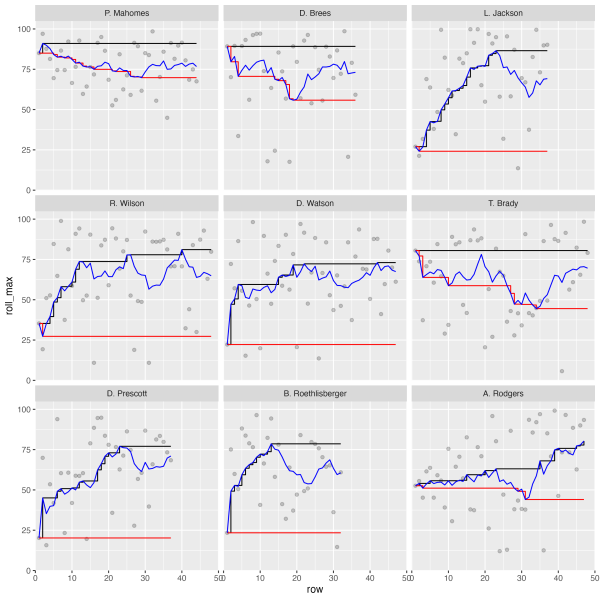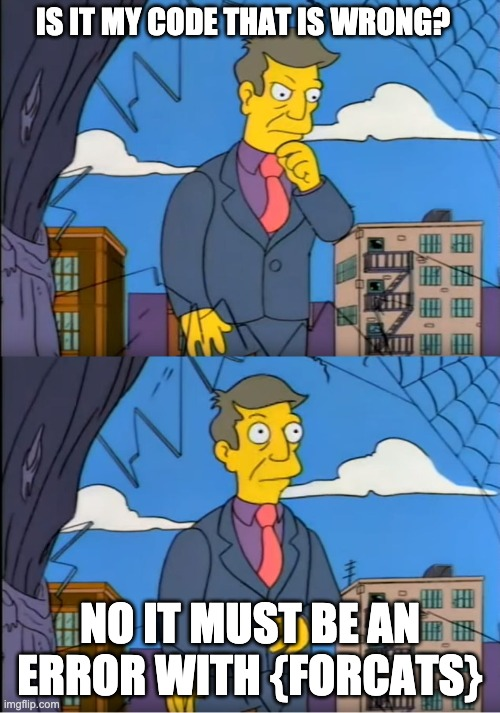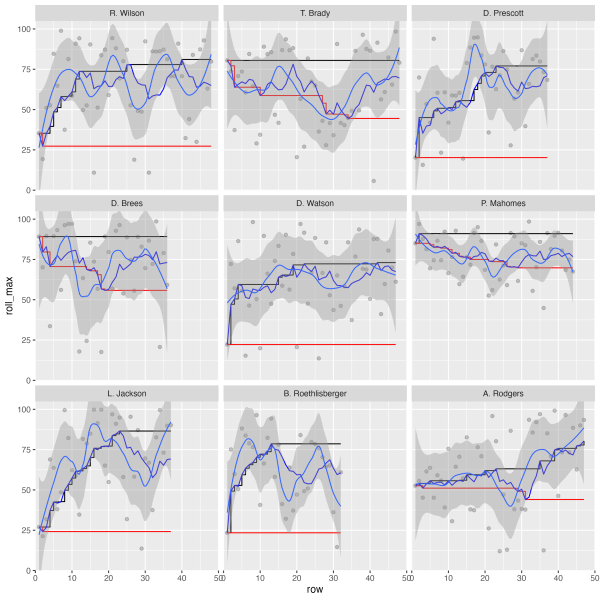# Reminder to test with a reprex

“It’s basically the rubber duck in disguise” - Jenny Bryan.

07-28-2021

It’s been a while since my last post, so I wanted to get back into the swing of things with a shorter article. My goal was to get a new one up on the blog before August 1st.

As such, I was working late on a much longer form article and as part of this I wanted to display some smoothed lines for several players on one plot. A sneak peek of some of this below:Now, I’ve been programming in R for quite some time, but for the life of me I couldn’t get my facets to align properly. This led to a bit of frustration as I “knew” what I was doing should be right but wasn’t returning the expected “correct” output.

This article walks through why I believe `{reprex}` driven testing is so useful for compartmentalizing a problem and getting at the specifics of why our expectation sometimes don’t match the output.

Like most useful ideas, I’m far from the first person to think of it!

I’ve decided that preparing a reprex is, in fact, a very effective technique for solving your own problems

it’s basically the rubber duck in disguise

— Jenny Bryan (@JennyBryan) January 4, 2018

My problem-solving workflow:

1. 🤬 something’s wrong with this pkg
2. I’m gonna open a GitHub issue
3. Wait, I need to add a reprex
4. Oohhhhhh 💡
5. Problem solved ✅ https://t.co/8GVJvR71YL

— Garrick Aden-Buie (@grrrck) June 19, 2021

Software engineering research question: if people are required to create a short reproducible example (reprex) when filing a bug, how often does doing that result in them solving their own problem?

— Greg Wilson (@gvwilson) February 22, 2021

That’s a few folks who I have a great deal of respect for, and as usual they have great advice for us! (Write a `reprex` and you’ll likely solve the problem as you go)

## Who’s problem is this?

Now whenever I run into errors, mistakes, or unexpected outputs I ask myself:

Is this a “Tom” problem? Or is this R’s fault?Principle skinner out of touch meme, with the text “Is it my code that is wrong?” and “No it must be an error with the forcats package” overlaid.

The short answer is that it’s almost always a “me” problem, as R is just diligently following the commands I gave to it. However, in some rare cases I do find a bug and can use the `{reprex}` testing I’ve done to quickly log a useful a bug report for the package developers.

If you haven’t run into the term “reprex” before, it is a:

“minimal reproducible example”

You can read more about the `{reprex}` package on the package documentation site.

If you’re asking for R help, reporting a bug, or requesting a new feature, you’re more likely to succeed if you include a good reprex.

That quote is from the `{reprex}` docs, and I’ll add to it that a reprex can help with you solving your own problems BEFORE even submitting the bug request!

## The Goal

While writing the article, I wanted to create a scatter plot, and facet the players by their respective QBR (Quarterback ratings) average scores. However, I kept getting the players to `facet_wrap()` by somewhat of an alphabetical order instead of in descending ranked order. This was a hint that my factor ordering via `{forcats}` was not being respected. I have included the “wrong” plot below.A 3x3 grid facetted by quarterback of the observation number on the X axis and the rolling max, min, and average on the Y-axis. The quarterbacks are facetted according to their alphabetical names.

This was of course frustrating, so I started doing some testing/troubleshooting of the complex plot as it was.

## Initiate testing

Let’s reproduce the initial problem. We’ll load the necessary packages, bring in the data, and generate the respective dataframes/plots as well. I’m intentionally leaving the code without comments and as a single code chunk as this is supposed to be a bit like a random code review. In retrospect the error is quite clear to me, but I was honestly stumped for a good chunk of time.

``````library(slider)
library(tidyverse)
library(espnscrapeR)

all_qbr <- crossing(season = 2018:2020, week = 1:17) %>%
pmap_dfr(get_nfl_qbr)

all_college_qbr <- 2018:2020 %>%
map_dfr(~get_college_qbr(.x, type = "weekly"))

test_data <- all_qbr %>%
select(player_id, qbr = qbr_total, team_abb, short_name) %>%
mutate(pure_avg = mean(qbr)) %>%
group_by(player_id) %>%
filter(n() >= 30) %>%
mutate(
short_name = fct_reorder(short_name, qbr, .fun= mean, .desc = TRUE),
grp_avg = mean(qbr, na.rm = TRUE),
diff_avg = qbr - pure_avg,
roll_mean = slide_dbl(qbr, mean, .before = 8L),
roll_max = slide_dbl(roll_mean, max, .before = Inf),
roll_min = slide_dbl(roll_mean, min, .before = Inf),
row = row_number()
) %>%
ungroup()

test_plot <- test_data %>%
filter(grp_avg >= 62) %>%
ggplot(aes(x = row, y = roll_max)) +
geom_point(aes(y = qbr), alpha = 0.2) +
geom_step() +
geom_step(aes(y = roll_min), color = "red") +
geom_line(aes(y = roll_mean), color = "blue") +
geom_smooth(aes(y = qbr),method = "loess", size = 0.5, span = 0.35) +
facet_wrap(~factor(short_name)) +
coord_cartesian(ylim = c(0, 105), xlim = c(0, 50), expand = FALSE)

test_plot
``````A 3x3 grid facetted by quarterback of the observation number on the X axis and the rolling max, min, and average on the Y-axis. The quarterbacks are facetted according to their alphabetical names.

### Maybe it’s a factor thing?

My initial thought is “it seems the factors are not being represented correctly”. Since I’m using `forcats::fct_reorder(.f = short_name, .x = qbr, .fun = mean, .desc = TRUE)` the Quarterback names should respect the “ranking” by their respective descending average QBR.

However, what I was getting out in `{ggplot2}` is ordered as below. It’s not in any real order!

``````test_data %>%
filter(grp_avg >= 62) %>%
distinct(short_name, grp_avg) %>%
arrange(short_name)
``````
``````# A tibble: 9 x 2
short_name        grp_avg
<fct>               <dbl>
1 R. Wilson            66.4
3 D. Prescott          62.9
4 D. Brees             71.4
5 D. Watson            63.4
6 P. Mahomes           77.2
7 L. Jackson           68.4
8 B. Roethlisberger    62.6
9 A. Rodgers           62.1``````

What I wanted, and how it “should” arrange like below:

``````test_data %>%
filter(grp_avg >= 62) %>%
mutate(short_name = fct_reorder(short_name, qbr, .fun = mean, .desc = TRUE)) %>%
distinct(short_name, grp_avg) %>%
arrange(short_name)
``````
``````# A tibble: 9 x 2
short_name        grp_avg
<fct>               <dbl>
1 P. Mahomes           77.2
2 D. Brees             71.4
3 L. Jackson           68.4
4 R. Wilson            66.4
5 D. Watson            63.4
7 D. Prescott          62.9
8 B. Roethlisberger    62.6
9 A. Rodgers           62.1``````

So let’s take a look at the `test_data` object. The factors are simply “wrong”! We know we’re looking for “P. Mahomes” to always lead our rankings in this dataset. The results below are not in any “real” order!

``````test_data %>%
filter(grp_avg >= 62) %>%
distinct(short_name) %>%
arrange(short_name) %>%
pull() %>%
levels()
``````
``````  "M. Ryan"           "M. Stafford"       "C. Newton"
 "A. Dalton"         "R. Tannehill"      "K. Cousins"
 "R. Wilson"         "D. Carr"           "T. Brady"
 "C. Wentz"          "D. Prescott"       "D. Brees"
 "M. Trubisky"       "J. Goff"           "B. Mayfield"
 "D. Watson"         "P. Mahomes"        "S. Darnold"
 "L. Jackson"        "K. Murray"         "J. Allen"
 "P. Rivers"         "B. Roethlisberger" "A. Rodgers"       ``````

I thought to try to correct it manually. I could just get a vector of the quarterbacks according to the ranking and then apply it with `factor(short_names, levels = qb_lvls)`. This gets us the “right” order of the names BUT it doesn’t really solve our underlying problem or tell us why it’s not working out as expected. This is a “bandaid” instead of a “cure” to my problem! If I did that I would never know if I had a wrong mental model, if there was an actual bug in `{ggplot2}` or `{forcats}` (unlikely), or if I was simply coding it all wrong.

``````qb_lvls <- test_data %>%
group_by(short_name) %>%
distinct(grp_avg) %>%
arrange(desc(grp_avg)) %>%
pull(short_name) %>%
as.character()

qb_lvls
``````
``````  "P. Mahomes"        "D. Brees"          "L. Jackson"
 "R. Wilson"         "D. Watson"         "T. Brady"
 "D. Prescott"       "B. Roethlisberger" "A. Rodgers"
 "M. Ryan"           "M. Stafford"       "J. Allen"
 "P. Rivers"         "K. Murray"         "R. Tannehill"
 "K. Cousins"        "B. Mayfield"       "D. Carr"
 "J. Goff"           "C. Wentz"          "M. Trubisky"
 "A. Dalton"         "C. Newton"         "S. Darnold"       ``````

### Make a reprex

So far, I dove into a few troubleshooting steps but it didn’t clarify what my underlying problem was. What I should have done was create a reprex or break the problem down into smaller pieces! I was about to ask for some help, so I wanted to generate a `reprex` to use as the “ask”.

Now for the `reprex`, I don’t want to include data scraped from the internet as the data doesn’t really matter (and it requires a separate package - `espnscrapeR`). So let’s simulate data!

``````library(tidyverse)

set.seed(20210728)

sim_data <- crossing(
grp = letters[1:4],
series = 1:10
) %>%
mutate(
val = rnorm(n = nrow(cur_data()), mean = 70, sd = 8)
)

sim_data
``````
``````# A tibble: 40 x 3
grp   series   val
<chr>  <int> <dbl>
1 a          1  63.5
2 a          2  82.0
3 a          3  75.6
4 a          4  64.8
5 a          5  72.7
6 a          6  74.2
7 a          7  75.0
8 a          8  70.3
9 a          9  69.2
10 a         10  69.7
# … with 30 more rows``````

We also don’t need ALL the calculations we are doing, so let’s simplify it to just the minimal calculation:
- a grouped average
- an ordered factor according to that grouped average

``````sim_plot_df <- sim_data %>%
group_by(grp) %>%
mutate(
grp_avg = mean(val, na.rm = TRUE),
grp_fct = fct_reorder(grp, val, .fun = mean, .desc = TRUE)
) %>%
ungroup()

sim_plot_df
``````
``````# A tibble: 40 x 5
grp   series   val grp_avg grp_fct
<chr>  <int> <dbl>   <dbl> <fct>
1 a          1  63.5    71.7 a
2 a          2  82.0    71.7 a
3 a          3  75.6    71.7 a
4 a          4  64.8    71.7 a
5 a          5  72.7    71.7 a
6 a          6  74.2    71.7 a
7 a          7  75.0    71.7 a
8 a          8  70.3    71.7 a
9 a          9  69.2    71.7 a
10 a         10  69.7    71.7 a
# … with 30 more rows``````

Let’s check what the order should be - looks like `c > a > d > b`

``````sim_plot_df %>%
distinct(grp, grp_avg) %>%
arrange(desc(grp_avg))
``````
``````# A tibble: 4 x 2
grp   grp_avg
<chr>   <dbl>
1 c        72.7
2 a        71.7
3 d        71.0
4 b        67.4``````

Now, let’s confirm what are the returned levels?

``````sim_plot_df %>%
pull(grp_fct) %>%
levels()
``````
`` "a" "b" "c" "d"``

Our `reprex` returned the “wrong” values! At this point, I thought I had legitimately found a bug…but I wanted to go one step further and check the plotting. I went ahead and added back in some complexity to check myself - specifically adding a horizontal line to indicate the group average and a label of the actual numeric group average on each facet.

STILL WRONG!

``````sim_plot_df %>%
ggplot(aes(x = series, y = val)) +
geom_point() +
geom_hline(aes(yintercept = grp_avg), color = "red") +
geom_text(aes(x = 2.5, y = 100, label = round(grp_avg, digits = 1))) +
facet_wrap(~grp_fct, ncol = 4)
``````Last step was to confirm I could get `{ggplot2}` to return the expected behavior of `c > a > d > b`. I could get the “right” behavior by adding in the `fct_reorder()` across the overall data inside `facet_wrap()`.

``````sim_plot_df %>%
ggplot(aes(x = series, y = val)) +
geom_point() +
geom_smooth() +
geom_hline(aes(yintercept = grp_avg), color = "red") +
geom_text(aes(x = 2.5, y = 100, label = round(grp_avg, digits = 1))) +
facet_wrap(~fct_reorder(grp_fct, val, .fun = mean, .desc = TRUE), ncol = 4)
``````At this point the answer stared me right in the face!

I could get the “right” behavior by adding in the `fct_reorder()` across the overall data inside `facet_wrap()`.

Emphasis there added on “overall data”. In my `group_by` + `mutate` I was applying a factor transformation WITHIN each group so each factor was basically a factor with only 1 level (the specific group), and when combined back into the dataframe it returned an overall factor with levels simply according to the order of the rows in the data.

Here’s a peek at the initial `reprex`.

``````sim_plot_df <- sim_data %>%
group_by(grp) %>%
mutate(
grp_avg = mean(val, na.rm = TRUE),
# this should be done BEFORE grouping
grp_fct = fct_reorder(grp, val, .fun = mean, .desc = TRUE)
) %>%
ungroup()
``````

and now after I’ve moved the `fct_reorder()` ahead of the `group_by()`.

``````sim_plot_df_fixed <- sim_data %>%
# fct_reorder done BEFORE grouping
mutate(grp_fct = fct_reorder(grp, val, .fun = mean, .desc = TRUE)) %>%
group_by(grp) %>%
mutate(
grp_avg = mean(val, na.rm = TRUE)
) %>%
ungroup()

sim_plot_df_fixed %>%
ggplot(aes(x = series, y = val)) +
geom_point() +
geom_hline(aes(yintercept = grp_avg), color = "red") +
geom_text(aes(x = 2.5, y = 100, label = round(grp_avg, digits = 1))) +
facet_wrap(~fct_reorder(grp_fct, val, .fun = mean, .desc = TRUE), ncol = 4)
``````By breaking the problem down into the simplest version of itself, and carefully checking my assumptions as I went, I was able to get at the root of the problem.

In essence by preparing the `reprex` in preparation of asking for help, I was able to solve the problem.

In the words of Jenny Bryan:

it’s basically the rubber duck in disguiseA Giant floating yellow rubber duck in the ocean outside Hong Kong

So go out, create your `reprex`, solve some of your own problems, and submit even better bug reports!

### Citation

`Mock (2021, July 28). The Mockup Blog: Reminder to test with a reprex. Retrieved from https://themockup.blog/posts/2021-07-28-reminder-to-test-with-a-reprex/`
```@misc{mock2021reminder,